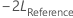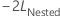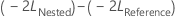# Specify the default settings for Fit Mixed Effects Model

Tools > Options > Linear Models > Mixed Effects Model

Specify the estimation method, test method for fixed effect terms, and results to display. The changes you make to the defaults remain until you change them again, even after you exit Minitab.

Estimation method

You can select either Restricted maximum likelihood (REML) or Maximum likelihood (ML). Usually, you use Restricted maximum likelihood (REML) because the variance component estimator by REML is approximately unbiased, while the ML estimator is biased. However, the bias gets smaller for larger sample sizes.

Use Maximum likelihood (ML) if you need to test whether a nested model with a fewer number of fixed effect terms is as good as its corresponding reference model that has more fixed effect terms, given both models have the same number of random terms and error variance structure. Specifically, letbe the negative 2 log likelihood from the full model, andbe the negative 2 log likelihood from the smaller model.

Under the null hypothesis, asymptotically,follows a chi- square distribution with degrees of freedom equal to the difference in the number of fixed effect terms between the reference model and the nested model. You can use the likelihood ratio test to evaluate whether a subset of fixed effect terms can be removed from the reference model.

For more information on the likelihood ratio test of fixed parameters in a mixed effects model, see B. T. West, K.B. Welch, and A.T. Gałecki (2007). Linear Mixed Models: A Practical Guide Using Statistical Software, First Edition. Chapman and Hall/CRC (34–36).

Test method for fixed effects

Usually, you use Kenward-Roger approximation because the calculations include an adjustment that reduces bias from small sample sizes. You can also use Satterthwaite approximation. In general, the larger the sample size, the less difference between the two methods.

Display of results
Factor information
Display a summary of the factor names, whether the factors are random, the numbers of levels, and the levels.
Iteration history
Displays the number of iterations until the algorithm converges and the value of the –2 log likelihood at each iteration.
Variance components
Display the estimates of the variance components.
Variance-covariance matrix of variance component estimates
Display the variance-covariance matrix of the variance component estimates.
Fixed effect tests
Display hypothesis tests about whether the means at different levels of the fixed effects are equal.
Random effect predictions
Display statistics for the random terms in the model including the best linear unbiased prediction (BLUP) for the level effect of a random term.
Marginal fitted equation
Display the equation for the marginal fitted values. The marginal fits represent the mean responses at the combined levels of the fixed factors with the entered covariate values (if any). The calculations for marginal fits do not use random factor levels.
• Separate equation for each set of factor levels: Display a separate equation for each combination of factor levels.
• Single equation: Display one equation that includes all levels of all factors.
Conditional fitted equation
Display the equation for the conditional fitted values. The conditional fits are the fitted values for the levels of the random factors in the data. Use the conditional fits to explore differences among the specific levels of the random factors in the study.
• Separate equation for each set of factor levels: Display a separate equation for each combination of factor levels.
• Single equation: Display one equation that includes all levels of all factors.
Marginal fits and diagnostics
• Only for unusual observations: Display the marginal fitted values, the marginal residuals, and the diagnostic statistics for only the unusual observations.
• For all observations: Display the marginal fitted values, the marginal residuals, and the diagnostic statistics for all observations.
Conditional fits and diagnostics
• Only for unusual observations: Display the conditional fitted values, the residuals, and the diagnostic statistics for only the unusual observations.
• For all observations: Display the conditional fitted values, the residuals, and the diagnostic statistics for all observations.
By using this site you agree to the use of cookies for analytics and personalized content.  Read our policy